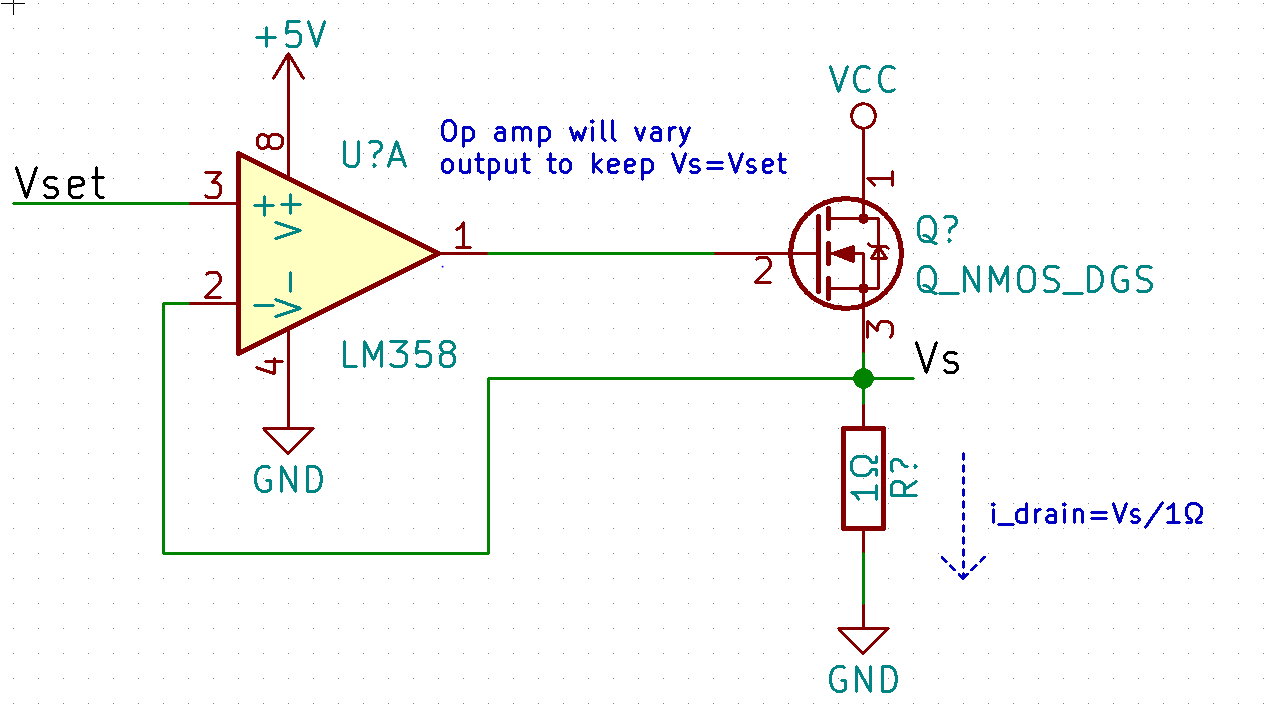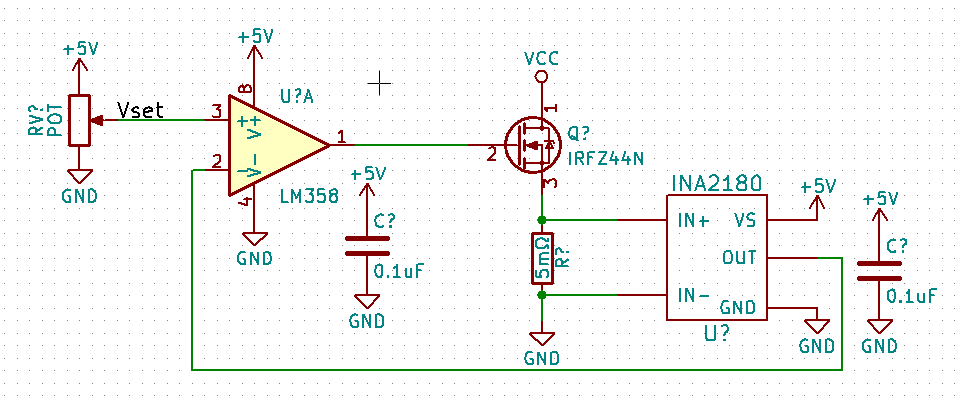I’m starting a new project series, I am going to call it Mini Circuits! Basically my idea is to make some circuits for some pretty common situations I have come across at work, and make a dozen or so. They won’t be super advanced circuits, but hopefully will provide useful in the future. So let’s begin.

# Mini Circuit #1: Constant Current Sink

First step as always, design parameters.

• 1V to 40V operation
• 0A to 10A sink, with 0.01A resolution
• Thermal management

So this is a pretty simple circuit. I just recently watched EEVblog’s video about his Constant Current Load so my design will be inspired by that. Let’s begin with picking the most important part, the MOSFET. I am going with the IRFZ44N from Infineon Technologies – it matches the specs I need without costing much. I’m still not too great at picking components, so let’s just start the design with this MOSFET.

So the basic constant current circuit with a mosfet is shown below:The picture hopefully explains itself. There is a bit of a problem here, however. At 10A, the voltage across the sense resistor would need to be 10V – we want to be able to sink 10A at 1V max, so that would require a 1V/10A = .1Ω resistor MAX. At lower currents (let’s say 1A) the voltage Vset would need to be capable of precisely setting that voltage, and etc etc etc. It is much better using an amplifier with a super small sense resistor. So let’s do some digging.

The INA2180 is a current sense amplifier from Texas Instruments. If we use that with a 5mΩ sense resistor, using the equation in the datasheet we can calculate the max power dissipation required as P = R * I^2 = 0.005 * 10^2 = 0.5W. It turns out that there are a BUNCH of >0.5W 5mΩ SMD resistors on Digikey. Back to the 2180, it is available in 4 different gains: 20V/V, 50V/V, 100V/V, and 200V/V. At 5mΩ with 0.01A of current (our minimum resolution) this results in .005Ω0.01A = 50uV. Multiplied by a gain of 100V/V gives us 5mV. The drift and inaccuracies in the op amp powering the MOSFET may have issues this low, but ah well. This circuit needs to be small and cheap. At 10A our voltage is 0.005Ω10A*100V/V = 5V. Perfect. So let’s look at the circuit.In future version I want to add a microcontroller, rotary encoder to replace the potentiometer, and an LCD for displaying the current. But let’s just test this circuit – this means I need to design the PCB and get it sent to be manufactured. I’ll post it below when I finish that up.

Mini Circuit 1: Constant Current Sink